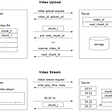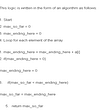# 879. Profitable Schemes

`class Solution:    def profitableSchemes(self, G: int, P: int, group: List[int], profit: List[int]) -> int:        # dp[g][p]        dp = [*(G+1) for i in range(P+1)]        dp = 1        for g, p in zip(group, profit):            for i in range(P, -1, -1):                for j in range(G-g, -1, -1):                    dp[min(P, i+p)][j+g] += dp[i][j]         return sum(dp[P])%(10**9+7)`

# 877. Stone Game

`const int N = 500 + 10;int dp[N][N];class Solution {public:    bool stoneGame(vector<int>& a) {        int n = a.size();        for (int i = 0; i < n; ++i) {            dp[i][i] = a[i];            dp[i][i] = -a[i];        }        for (int k = 1; k < n; ++k) {            for (int i = 0; i + k < n; ++i) {                int j = i + k;                dp[i][j] = max(a[i] + dp[i + 1][j], a[j] + dp[i][j - 1]);                dp[i][j] = min(-a[i] + dp[i + 1][j], -a[j] + dp[i][j - 1]);            }        }        return dp[n - 1] > 0;    }};`

# 873. Length of Longest Fibonacci Subsequence

`class Solution:    def lenLongestFibSubseq(self, A: List[int]) -> int:        vals_from_idx_i = collections.defaultdict(set)        vals_from_idx_i[len(A)-1] = {A[-1]}        for i in range(len(A)-1, -1, -1):            vals_from_idx_i[i] = vals_from_idx_i[i+1] | {A[i]}        def dfs():            fin_res = 2            open_list = []            for i in range(len(A)-2):                for j in range(i+1, len(A)-1):                    new_a = A[i]+A[j]                     if new_a in vals_from_idx_i[j+1]:                        fin_res = 3                        idx = A[j+1:].index(new_a)                        open_list.append((A[j],new_a, j+idx+1, 3 ))            while open_list:                a,b, j, d = open_list.pop()                new_a = a+b                if new_a in vals_from_idx_i[j+1]:                    if d+1>fin_res:                        fin_res = d+1                    idx = A[j+1:].index(new_a)                    new_idx = j+idx+1                    # early pruning                    if d+1+(len(A)-1-new_idx)>fin_res:                        open_list.append((b, new_a, new_idx, d+1))            return fin_res        fin_res = dfs()        return fin_res if fin_res>2 else 0`
`class Solution:    def lenLongestFibSubseq(self, A: List[int]) -> int:        dp = collections.defaultdict(lambda : 2)        s = set(A)        for j in range(len(A)):            for i in range(j):                if A[j] - A[i] < A[i] and A[j] - A[i] in s:                    dp[A[i], A[j]] = dp[(A[j] - A[i], A[i])] + 1        return max(dp.values() or )`

# 5304. XOR Queries of a Subarray

`class Solution:    def xorQueries(self, arr: List[int], queries: List[List[int]]) -> List[int]:        # the maximum number 10^9 has 30 bits        dp = [*30 for _ in range(len(arr)+1)]        for i,a in enumerate(arr):            this_a = bin(a)[2:]            dp[i+1] = dp[i][:]            for j, c in enumerate(this_a[::-1]):                if c=='1':dp[i+1][-j-1] = dp[i][-j-1] + 1                            res = []        for a,b in queries:            if a==b:                res.append(arr[a])            else:                xor_res = ['1' if (dp[b+1][k]-dp[a][k])&1 else '0' for k in range(30)]                this_res = int(''.join(xor_res), 2)                res.append(this_res)        return res`

# 5306. Minimum Insertion Steps to Make a String Palindrome

`class Solution:    def minInsertions(self, s: str) -> int:        from functools import lru_cache        @lru_cache(None)        def dp(l,r):            if l>=r:return 0            if s[l]==s[r]:return dp(l+1, r-1)            return min(dp(l, r-1), dp(l+1, r))+1        return dp(0, len(s)-1)`

# hackerrank Coin change

`def getWays(n, c):    from functools import lru_cache    #top down    @lru_cache(None)    def dp(n, m):        if n < 0:return 0        if n == 0:return 1        if m < 0:return 0        # take this coin and not take this coin        # dp table        #n=4        #conins = [1 2 3]        #          m=1                   m=2                             m=3                 # n=4                                                       dp(1,2)+dp(4,1)        # n=3                            # n=2                                 # n=1   dp(0,0)+dp(1,-1)      dp(-1,1)+dp(1,0)              dp(-2, 2)+dp(1,1)         # dp(1,2) =   dp(-2, 2)+dp(1,1) =  dp(-2, 2)+dp(-1,1)+"dp(0,0)"+dp(1,-1) =0+0+1+0        # it means 1 + 3 which is 4        # dp(4,1) = dp(2,1) +dp(4,0) = dp(0,1)+dp(2,0)+dp(4,0) = dp(0,1)+dp(2,0)+dp(4,0)=1+1+1=3         # 2+2        # 2+1+1        # 1+1+1+1        return dp(n-c[m], m) + dp(n,m-1)    #for n in range(1,n+1):    #    for m in range(len(c)):    #        print(n,m, dp(n,m))    return dp(n, len(c)-1)`
`def getWays(n, c):    from functools import lru_cache    @lru_cache(None)    def count_make_change(n, m):        if n < 0:return 0        if n == 0:return 1        if m <= 0:return 0        return count_make_change(n-c[m-1], m) + count_make_change(n,m-1)    return count_make_change(n, len(c))`
`    counts = [*m for _ in range(n+1)]    counts = *m    def dp_bottom_up(n,m):        for j in range(m):            for i in range(1,n+1):                # take it                if i-c[j]>=0:                    counts[i][j] += counts[i-c[j]][j]                # do not take it                if j-1>=0:                    counts[i][j] += counts[i][j-1]        return counts[n][m-1]    return dp_bottom_up(n,len(c))`
`def dp_bottom_up(n, m):        counts =  * (n+1)        counts = 1        for i in range(m):            for j in range(c[i],n+1):                counts[j] += counts[j-c[i]]        return counts[n]    return dp_bottom_up(n, len(c))`

# 823. Binary Trees With Factors

`class Solution:    def numFactoredBinaryTrees(self, A: List[int]) -> int:        dp = {}        for a in sorted(A):            dp[a] = sum(dp[b] * dp.get(a // b, 0) for b in dp if a % b == 0) + 1        return sum(dp.values()) % (10**9 + 7)`

# 818. Race Car

`class Solution:    def racecar(self, target: int) -> int:        def bfs():            from collections import deque            Q = deque([(0,1,0)])            seen = {(0,1)}            if target==0:return 0            while True:                p, s, d = Q.popleft()                if p==target:return d                for action in [True, False]:                    if action:                        this_p = p+s                        s*=2                        if this_p==target:return d+1                        if (this_p, s) not in seen:                            seen.add((this_p, s))                            Q.append((this_p, s,d+1))                    else:                        if s>0:s=-1                        else:s=1                        if (p,s) not in seen:                            seen.add((p, s))                            Q.append((p, s,d+1))                return bfs()`
`class Solution:    def __init__(self):         self.dp = {0: 0}            def racecar(self, t: int) -> int:        if t in self.dp: return self.dp[t]        n = t.bit_length()        if 2**n - 1 == t: self.dp[t] = n        else:            self.dp[t] = self.racecar(2**n - 1 - t) + n + 1            for m in range(n - 1):                self.dp[t] = min(self.dp[t], self.racecar(t - 2**(n - 1) + 2**m) + n + m + 1)        return self.dp[t]`
`class Solution:    def racecar(self, target: int) -> int:        def bfs():            from collections import deque            Q = deque([(0,1,0)])            print(0,1,0)            seen = {(0,1)}            if target==0:return 0            while True:                p, s, d = Q.popleft()                #print(p,s,d)    # True means A, False means R                for action in [True, False]:                    if action:                        print('A')                        this_p = p + s                        s <<= 1                        if this_p==target:                            print(this_p,s,d+1)                            return d+1                        if (this_p, s) not in seen:                            print(this_p,s,d+1)                            seen.add((this_p, s))                            Q.append((this_p, s,d+1))                    else:                        print('R')                        if s>0:s=-1                        else:s=1                        if (p,s) not in seen:                            print(p,s,d+1)                            seen.add((p, s))                            Q.append((p, s,d+1))                return bfs()`
`0 1 0A1 2 1R0 -1 1A3 4 2R1 -1 2A-1 -2 2RA7 8 3R3 -1 3A0 -2 3R1 1 3A-3 -4 3R-1 1 3A15 16 4R7 -1 4A2 -2 4R3 1 4A-2 -4 4`
`0 1 01 2 10 -1 13 4 21 -1 2-1 -2 27 8 33 -1 30 -2 31 1 3-3 -4 3-1 1 315 16 47 -1 42 -2 43 1 4-2 -4 42 2 4-7 -8 4-3 1 40 2 4-1 -1 431 32 515 -1 56 -2 57 1 50 -4 52 1 54 2 5-6 -8 5-2 1 54 4 52 -1 5-15 -16 5-7 1 5-2 2 5-3 -1 52 4 5-2 -2 563 64 631 -1 614 -2 615 1 64 -4 66 1 68 2 6-4 -8 63 2 66 4 64 -1 6-14 -16 6-6 1 6-1 2 6-2 -1 68 8 61 -2 6-31 -32 6-15 1 6-6 2 6-7 -1 60 4 6-4 -2 66 8 6-4 -4 6127 128 763 -1 730 -2 731 1 712 -4 714 1 716 2 70 -8 74 1 77 2 76 -1 710 4 78 -1 7-12 -16 7-4 1 75 4 710 8 73 -2 7-30 -32 7-14 1 7-5 2 7`
`-10 8-9 9-8 7-7 4-6 5-5 7-4 6-3 3-2 4-1 20 01 12 43 24 55 76 57 38 69 810 7`

# 813. Largest Sum of Averages

`from functools import lru_cacheclass Solution:    def largestSumOfAverages(self, a: List[int], k: int) -> float:        @lru_cache(None)        def dp(st, k):            if k == 1:return sum(a[st:])/(len(a)-st)            total, res = 0, -float('inf')            for i in range(st, len(a)-k+1):                total += a[i]                res = max(res, (total/(i-st+1)) + dp(i+1, k-1))            return res                return dp(0, k)`
`from functools import lru_cacheclass Solution:    def largestSumOfAverages(self, a: List[int], k: int) -> float:        cusum = list(itertools.accumulate(+a))        @lru_cache(None)        def dp(i, k):            if k == 1:return (cusum[-1]-cusum[i])/(len(a)-i)            return max((cusum[j+1]-cusum[i])/(j-i+1) + dp(j+1, k-1) for j in range(i, len(a)-k+1))                return dp(0, k)`
`from functools import lru_cacheclass Solution:    def largestSumOfAverages(self, a: List[int], k: int) -> float:        cusum = list(itertools.accumulate(+a))        @lru_cache(None)        def dp(i, k):            if i>=len(a):return 0            if k == 1:return (cusum[-1]-cusum[i])/(len(a)-i)            return max((cusum[j+1]-cusum[i])/(j-i+1) + dp(j+1, k-1) for j in range(i, len(a)))                return dp(0, k)`
`Runtime: 1260 ms, faster than 5.28% of Python3 online submissions for Largest Sum of Averages.Memory Usage: 12.9 MB, less than 100.00% of Python3 online submissions for Largest Sum of Averages.`
`class Solution:    def largestSumOfAverages(self, A: List[int], K: int) -> float:        dp=[[0 for j in range(K)] for i in range(len(A))]        for j in range(len(A)):            for i in range(K):                 if i==0:dp[j][i]=sum(A[:j+1])/len(A[:j+1])                else:                    if len(A[:j+1])<i+1:break                    for k in range(j):                        dp[j][i]=max(dp[k][i-1]+sum(A[k+1:j+1])/len(A[k+1:j+1]),dp[j][i])        return dp[-1][-1]`
`class Solution:    def largestSumOfAverages(self, a: List[int], k: int) -> float:        cusum = list(itertools.accumulate(+a))        N=len(a)        #dp[k] means from 0 to N-1 inclusively we have at most k groups        # dp[k] = maximum of below cases        #average(a[:1])+dp[k-1] from 1 to N-1 inclusively we have at most k-1 groups        #average(a[:2])+dp[k-1] from 2 to N-1 inclusively we have at most k-1 groups        #...        #average(a[:N-1])+dp[N-1][k-1] from N-1 to N-1 inclusively we have at most k-1 groups        # dp[k-1] from 1 to N-1 inclusively we have at most k-1 groups        # average(a[1:2])+dp[k-2]        # average(a[1:3])+dp[k-2]        # ...        # average(a[1:N-1])+dp[N-1][k-2]        # until now, the solution can be clear. We should maintain a 2D dp table        # dp[i][k] it means maximum average sum         #     from index i to N-1 inclusively, we have at most k groups        # we can do it bottom up or top down        # we can use cusum to speed up the average operation.        dp = [*(k+1) for i in range(len(a))]        # the very bottom case will be k is 1, it means we only have one group from i to N-1 inclusively        for at_most_group in range(1, k+1):            for begin_i in range(N):                if at_most_group == 1:                    dp[begin_i][at_most_group] = (cusum[-1]-cusum[begin_i])/(N-begin_i)                else:                    this_res = []                    for j in range(begin_i, N):                        # we divide at_most_group to 1+(at_most_group-1)                        # (at_most_group-1) is already caculated.                         # for eaxmple, dp means                         # we are dividing list a from index 3 to N-1 into at most 2 groups                        # if we pick up index 3 to 4 as first group, then we still need dp                        # dp means we begin from index 5, we need at most 1 group.                        # dp is already caculated as k is from smaller to larger.                        first_group_ave = (cusum[j+1]-cusum[begin_i])/(j-begin_i+1)                        if j+1<N:                            this_res.append(first_group_ave+dp[j+1][at_most_group-1])                        else:                            this_res.append(first_group_ave)                    dp[begin_i][at_most_group] = max(this_res)        # final result will be begin at 0 and at most it has k groups.        return dp[k]`

--

--

--

## More from Jimmy Shen

Data Scientist/MLE/SWE @takemobi

Love podcasts or audiobooks? Learn on the go with our new app.

## Recommended from Medium## My experience with crio.do’s learn software development by doing## Docker Cloud’s Swarm mode feature## Web Scraping Using Python — The Second Part## Road Map to become a python🐍🐍🐍👩🏻‍💻👨🏻‍💻 developer in 2020## Jimmy Shen

Data Scientist/MLE/SWE @takemobi

## More from Medium